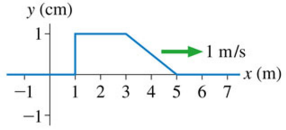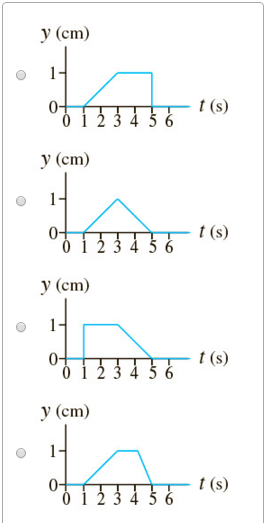# Problem: Problem 15.9(Figure 1) is a snapshot graph of a wave at t = 0 s.What is the history graph for this wave at x = 6 m, for t = 0 s to 6 s?

###### FREE Expert Solution
81% (233 ratings)View Complete Written Solution
###### Problem Details

Problem 15.9

(Figure 1) is a snapshot graph of a wave at t = 0 s.What is the history graph for this wave at x = 6 m, for t = 0 s to 6 s?